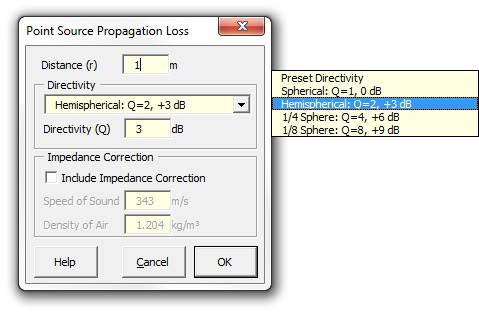### Strutt Help

Point Source Propagation Loss    1/1, 1/3

Strutt|Propagation|Point Source provides the correction for spherical sound radiation from a source, given the distance from the source (r) in metres. A directivity index (in dB) is used to allow correction for hemispherical, 1/4 sphere and 1/8 sphere radiation, either directly entered by the user or via the drop-down menu provided.Strutt implements the correction as:

L_p=L_W-20log(r)-11+DI

The user may select to include the full propagation term including a correction for the specific acoustic impedance rho c
(:p^2:)=(W rho c)/(4 pi r^2)
L_p=L_W-20log(r)-11+10*log(rho c W_0 / p_0^2) + DI

For typical conditions, 10log(rho c W_0 / p_0^2)~=0.1 dB and may be neglected; however Strutt allows the impedance term to be included if calculations are conducted under non-standard conditions.